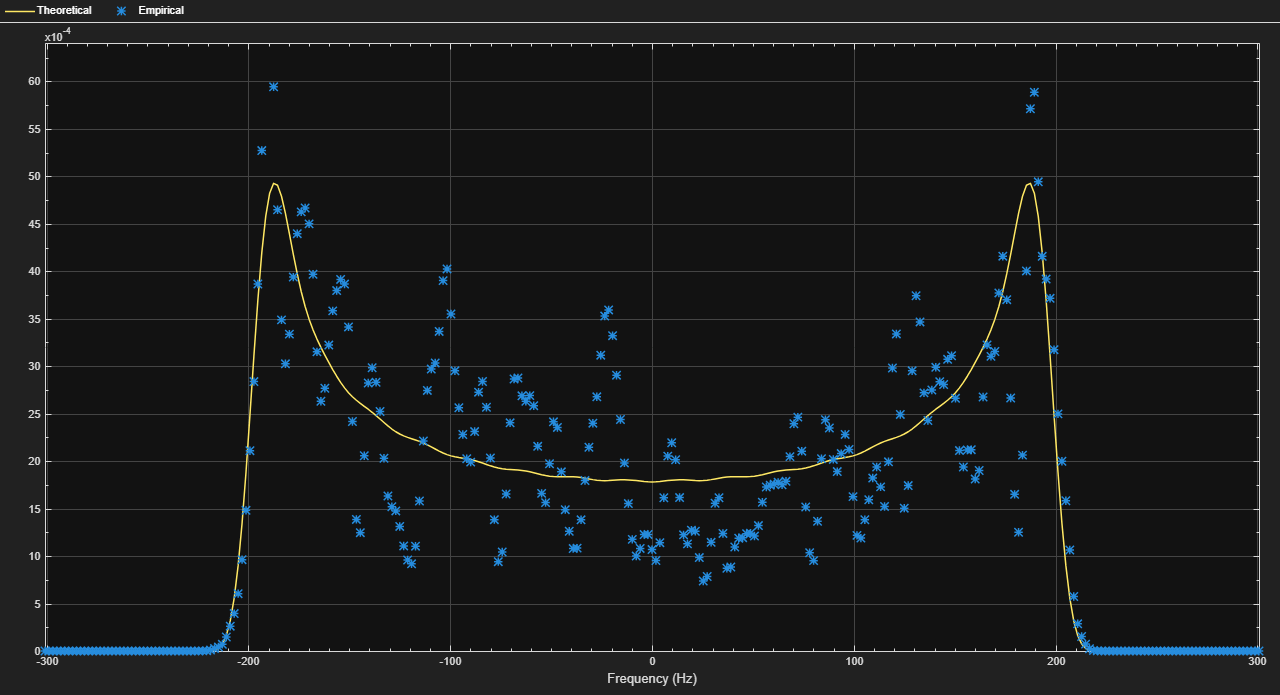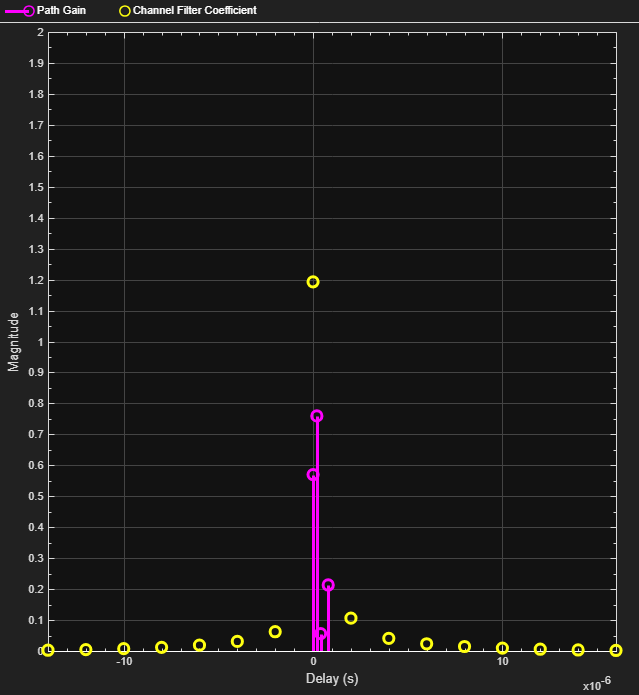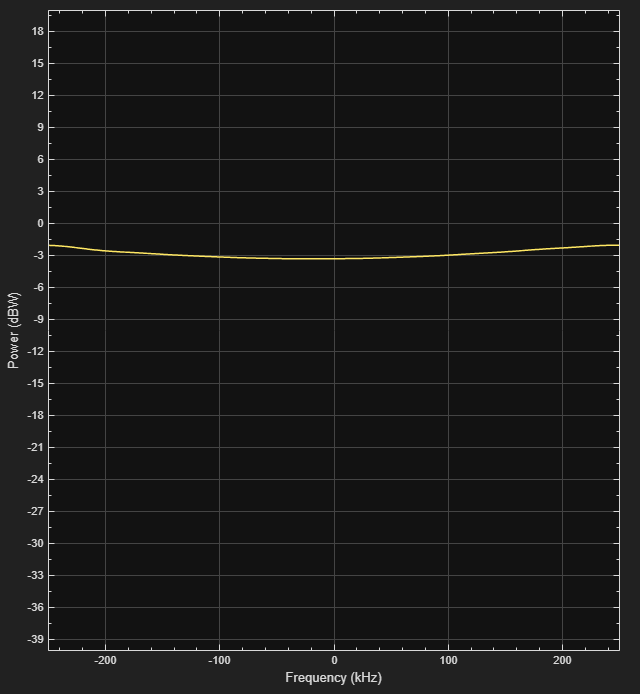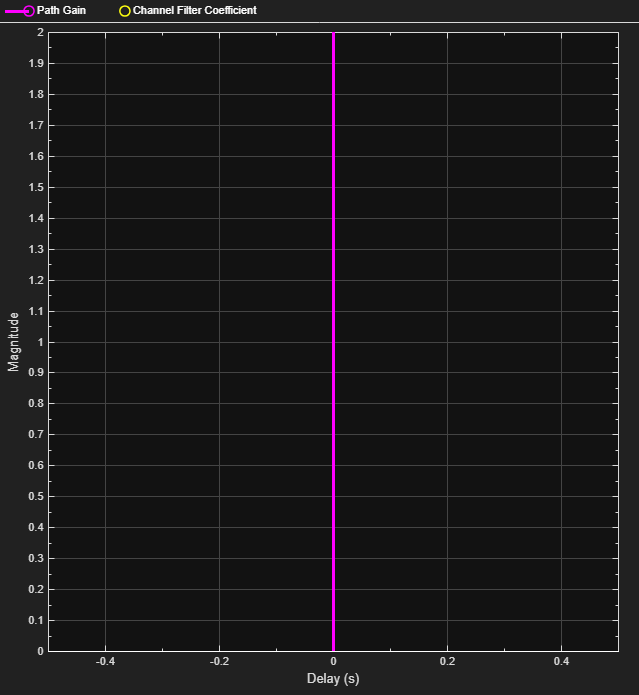Documentation

### This is machine translation

Mouseover text to see original. Click the button below to return to the English version of the page.

This model shows how to use the SISO Fading Channel block from the Communications Toolbox™ to simulate multipath Rayleigh and Rician fading channels, which are useful models of real-world phenomena in wireless communications. These phenomena include multipath scattering effects, time dispersion, and Doppler shifts that arise from relative motion between the transmitter and receiver. The model also shows how to visualize channel characteristics such as the impulse and frequency responses, Doppler spectrum and component gains.

### Model and Parameters

The example model simulates QPSK transmission over a multipath Rayleigh fading channel and a multipath Rician fading channel. Both the channel blocks are configured from the SISO Fading Channel library block. You can control transmission and channel parameters via workspace variables.

```modelname = 'commmultipathfading'; rayleighBlock = [modelname '/Rayleigh Channel']; ricianBlock = [modelname '/Rician Channel']; rayleighCD = [modelname '/Rayleigh Constellation Diagram']; pathGainBlock = [modelname '/Path Gains (dB)']; open_system(modelname); ```The following variables control the "Bit Source" block. By default, the bit rate is 10M b/s (5M sym/s) and each transmitted frame is 2000 bits long (1000 symbols).

```bitRate % Transmission rate (b/s) bitsPerFrame % Number of bits per frame ```
```bitRate = 10000000 bitsPerFrame = 2000 ```

The following variables control both the Rayleigh and Rician fading channel blocks. By default, the channels are modeled as four fading paths, each representing a cluster of multipath components received at around the same delay.

```delayVector % Discrete path delays (s) gainVector % Average path gains (dB) ```
```delayVector = 1.0e-06 * 0 0.2000 0.4000 0.8000 gainVector = 0 -3 -6 -9 ```

By convention, the delay of the first path is typically set to zero. For subsequent paths, a 1 microsecond delay corresponds to a 300 m difference in path length. In some outdoor multipath environments, reflected paths can be up to several kilometers longer than the shortest path. With the path delays specified above, the last path is 240 m longer than the shortest path, and thus arrives 0.8 microseconds later.

Together, the path delays and average path gains specify the channel's delay profile. Typically, the average path gains decay exponentially with delay (i.e., the dB values decay linearly), but the specific delay profile depends on the propagation environment. On each channel block, we have also turned on the option to normalize the average path gains so that their average gain is 0 dB over time.

The following variable controls the maximum Doppler shift which is computed as v*f/c, where v is the mobile speed, f is the carrier frequency, and c is the speed of light. The default maximum Doppler shift in the model is 200 Hz which corresponds to a mobile speed of 65 mph (30 m/s) and a carrier frequency of 2 GHz.

```maxDopplerShift % Maximum Doppler shift of diffuse components (Hz) ```
```maxDopplerShift = 200 ```

The following variables apply to the Rician fading channel block. The Doppler shift of the line-of-sight component is typically smaller than the maximum Doppler shift (above) and depends on the mobile's direction of travel relative to the direction of the line-of-sight path. The K-factor specifies the ratio of average received power from the line-of-sight path relative to that of the associated diffuse components.

```LOSDopplerShift % Doppler shift of line-of-sight component (Hz) KFactor % Ratio of specular power to diffuse power (linear) ```
```LOSDopplerShift = 100 KFactor = 10 ```

The SISO Fading Channel block can visualize channel impulse response, frequency response, and Doppler spectrum while the model is running. To invoke it, set the `Channel visualization` parameter to the desired channel characteristic(s) before running the model. Note that turning on channel visualization may slow down your simulation.

By default, the channel's delay span (0.8 microseconds) is larger than the input QPSK symbol period (0.2 microseconds), which causes considerable intersymbol interference (ISI). So the resultant channel frequency response is not flat and may have deep fades over the 10M Hz signal bandwidth. Because the power level varies over the bandwidth, it is referred to as frequency-selective fading.

Setting the channel block's `Channel visualization` parameter to 'Impulse response' shows the bandlimited impulse response (yellow circles). The visualization also shows the delays and magnitudes of the underlying fading path gains (pink stems) clustered around the peak of the impulse response. Note that the path gains do not equal the `Average path gains (dB)` parameter value because the Doppler effect causes the gains to fluctuate over time.

```set_param(rayleighBlock, 'Visualization', 'Impulse response'); set_param(modelname, 'SimulationCommand', 'start'); set_param(modelname, 'SimulationCommand', 'pause'); ```As displayed, the channel impulse response coincides with the path gains for this delay profile because the discrete path delays are all integer multiples of the input symbol period. In this case, there is also no channel filter delay.

Similarly, setting the `Channel visualization` parameter to 'Frequency response' shows the channel's frequency response. You can also set `Channel visualization` to 'Impulse and frequency responses' to display both impulse and frequency responses side by side. You can see that the channel's power level varies across the whole bandwidth.

```set_param(modelname, 'SimulationCommand', 'stop'); set_param(rayleighBlock, 'Visualization', 'Frequency response'); set_param(rayleighBlock, 'SamplesToDisplay', '50%'); set_param(modelname, 'SimulationCommand', 'start'); set_param(modelname, 'SimulationCommand', 'pause'); ```As shown above, you can also control the percentage of the input samples to be visualized by changing the channel block's `Percentage of samples to display` parameter. In general, the smaller the percentage, the faster the model runs. Once the visualization figure opens, click the `Playback` button and turn off the `Reduce Updates to Improve Performance` or `Reduce Plot Rate to Improve Performance` option to further improve display accuracy. The option is on by default for faster simulation. To see the channel response for every input sample, uncheck this option and set `Percentage of samples to display` to '100%'.

For the same channel specification, we now display the Doppler spectrum for its first discrete path, which is a statistical characterization of the fading process. The channel block makes periodic measurements of the Doppler spectrum (blue stars). Over time with more samples processed by the block, the average of this measurement better approximates the theoretical Doppler spectrum (yellow curve).

```set_param(modelname, 'SimulationCommand', 'stop'); set_param(rayleighBlock, 'Visualization', 'Doppler spectrum'); set_param(modelname, 'StopTime', '3'); set_param(modelname, 'SimulationCommand', 'start'); set_param(modelname, 'SimulationCommand', 'pause'); while get_param(modelname, 'SimulationTime') < 2 set_param(modelname, 'SimulationCommand', 'continue'); pause(1); set_param(modelname, 'SimulationCommand', 'pause'); end ```By opening the constellation diagram following the Rayleigh channel block, you can see the impact of wideband fading on the signal constellation. To slow down the channel dynamics for visualization purposes, we reduce the maximum Doppler shift to 5 Hz. Compared with the QPSK channel input signal, you can observe obvious distortion in the channel output signal, due to the ISI from the time dispersion of the wideband signal.

```set_param(modelname, 'SimulationCommand', 'stop'); maxDopplerShift = 5; set_param(rayleighBlock, 'Visualization', 'Off'); set_param(rayleighCD, 'openScopeAtSimStart', 'on') sim(modelname, 0.2); ```When the bandwidth is too small for the signal to resolve the individual components, the frequency response is approximately flat because of the minimal time dispersion and very small ISI from the impulse response. This kind of multipath fading is often referred to as narrowband fading, or frequency-flat fading.

To observe the effect, we now reduce the signal bandwidth from 10M b/s (5M sym/s) to 1M b/s (500K sym/s), so the channel's delay span (0.8 microseconds) is much smaller than the QPSK symbol period (2 microseconds). Effectively, all delayed components combine at a single delay (in this case, at zero).

```bitRate = 1e6 % 50 kb/s transmission ```
```bitRate = 1000000 ```

We can visually validate this narrowband fading behavior by setting the `Channel visualization` parameter to 'Impulse and frequency responses' for the Rayleigh channel block and then running the model.

```close_system(rayleighCD); set_param(rayleighCD, 'openScopeAtSimStart', 'off') maxDopplerShift = 200; % Change back to the original value set_param(rayleighBlock, 'Visualization', 'Impulse and frequency responses'); set_param(modelname, 'SimulationCommand', 'start'); set_param(modelname, 'SimulationCommand', 'pause'); ```To simplify and speed up simulation, narrowband fading channels are often modeled as a single-path fading channel. That is, a multiple-path fading model overspecifies a narrowband fading channel. The following settings correspond to a narrowband fading channel with a completely flat frequency response.

```set_param(modelname, 'SimulationCommand', 'stop'); delayVector = 0; % Single fading path with zero delay gainVector = 0; % Average path gain of 0 dB set_param(modelname, 'SimulationCommand', 'start'); set_param(modelname, 'SimulationCommand', 'pause'); ```We now return to our original four-path fading channel and observe how narrowband fading causes signal attenuation and phase rotation, by opening the constellation diagram following the Rayleigh channel block. In addition to attenuation and rotation, you can see some signal distortion because of the small amount of ISI in the channel output signal. The distortion is far less than that seen above for a wideband channel.

```set_param(modelname, 'SimulationCommand', 'stop'); delayVector = [0 2 4 8]*1e-7; % Change back to original value gainVector = (0:-3:-9); % Change back to original value maxDopplerShift = 5; % Reduce to slow down channel dynamics set_param(rayleighBlock, 'Visualization', 'Off'); set_param(rayleighCD, 'openScopeAtSimStart', 'on') sim(modelname, 0.15); ```

```delayVector = 0; % Single fading path with zero delay gainVector = 0; % Average path gain of 0 dB maxDopplerShift = 200; % Change back to the original value close_system(rayleighCD); set_param(rayleighCD, 'openScopeAtSimStart', 'off') set_param(pathGainBlock, 'OpenAtSimulationStart', 'on'); sim(modelname, 0.1); ``````% Cleanup close_system(modelname, 0); ```